## FUZZYTHRESH

 Automatically thresholds an image to binary (b/w) format using the fuzzy c-means technique.

 USAGE: fuzzythresh [-r radius] [-g graph] infile outfile USAGE: fuzzythresh [-help] -r ..... radius ......... radius for spatial correlation; float; ......................... radius>=0; the default=0 signifies to ......................... ignore spatial correlation -g ..... graph .......... graph specifies whether to generate a ......................... histogram graph image displaying the ......................... location and value of the threshold; ......................... choices are: view or save; ......................... default is no graph PURPOSE: To automatically thresholds an image to binary (b/w) format using the fuzzy c-means technique. DESCRIPTION: FUZZYTHRESH automatically thresholds an image to binary (b/w) format. It assume the histogram is bimodal, i.e. is the composite of two bell-shaped distributions representing the foreground and background classes. The fuzzy c-means appoach iteratively thresholds the image, computes a weighted mean for the foreground (above threshold data) and background (at and below threshold value), computes a new threshold equal to the average of these two means and repeats until there is no change in threshold between successive iterations. The weighting factors are the normalized inverse square difference between each pixel in the foreground or background data sets and the corresponding mean. However, to allow for spatial correlation of the graylevels among neighboring pixels, a Gaussian filtered version of the weighting factors may be used. This script is similar to the isodatathresh script, except uses a weighted mean calculation. ARGUMENTS: -r radius ... RADIUS is the radius of a Gaussian (blur) filter to apply to the weighting factors for the mean calculation. This permits spatial correlation of the graylevels among neighboring pixels and is useful to remove noise from the result. The radius value should be set to the size of the features in the image. Values are floats greater than zero. The default=0 signifies to ignore spatial correlation. -g graph ... GRAPH specifies whether to generate a graph (image) of the histogram, displaying the location and value of the threshold. The choices are: view, save and none. If graph=view is selected, the graph will be created and displayed automatically, but not saved. If graph=save is selected, then the graph will be created and saved to a file using the infile name, with "_histog_fuzzy.gif" appended, but the graph will not be displayed automatically. If -g option is not specified, then no graph will be created. NOTE: It is highly recommended that the output not be specified as a JPG image as that will cause compression and potentially a non-binary (i.e. a graylevel) result. GIF is the recommended output format. CAVEAT: No guarantee that this script will work on all platforms, nor that trapping of inconsistent parameters is complete and foolproof. Use At Your Own Risk.

### EXAMPLES

 Fuzzy C-Means Thresholding Of Various Images -- Radius=0 Pictures Were Obtained from: blood.jpg from http://www.istanbul.edu.tr/eng/ee/jeee/main/pages/issues/is62/62008.pdf fingerprint.jpg from http://www.istanbul.edu.tr/eng/ee/jeee/main/pages/issues/is62/62008.pdf flower.jpg from http://www.jhlabs.com/ip/blurring.html house.jpg from http://en.wikipedia.org/wiki/Otsu's_method kanji.jpg from http://www.measurement.sk/2004/S1/Yong.pdf parts.gif from http://www.ph.tn.tudelft.nl/Courses/FIP/noframes/fip-Segmenta.html rice.jpg from http://www.istanbul.edu.tr/eng/ee/jeee/main/pages/issues/is62/62008.pdf tank.jpg from http://stinet.dtic.mil/cgi-bin/GetTRDoc?AD=ADA464347&Location=U2&doc=GetTRDoc.pdf textsample.jpg from http://signal.ece.utexas.edu/seminars/dsp_seminars/01fall/211_seeger_mf.pdf lena2g_edge1.jpg was created using the IM function -edge 1 Original Image Thresholded Image Histogram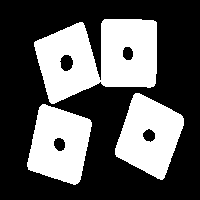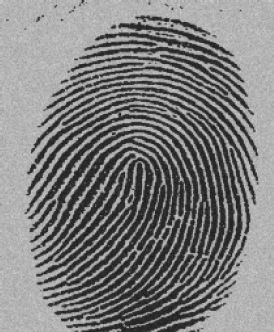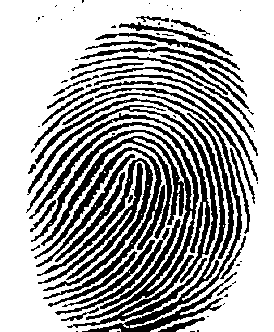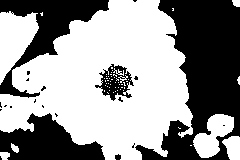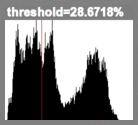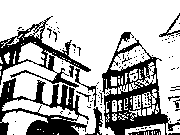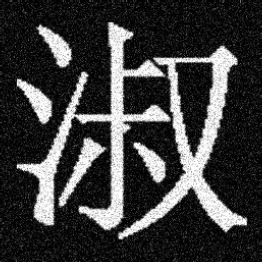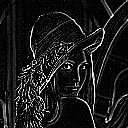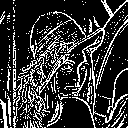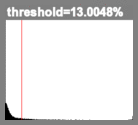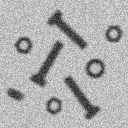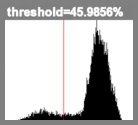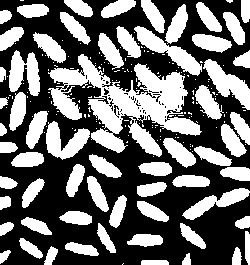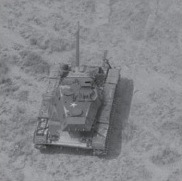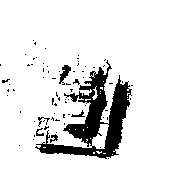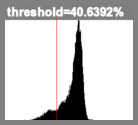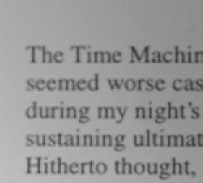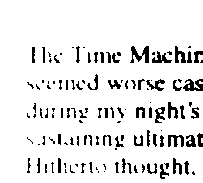Fuzzy C-Means Thresholding Of Various Images -- Radius>0 Pictures Were Obtained from: kanji.jpg from http://www.measurement.sk/2004/S1/Yong.pdf rice.jpg from http://www.istanbul.edu.tr/eng/ee/jeee/main/pages/issues/is62/62008.pdf lena2g_edge1.jpg was created using the IM function -edge 1 Original Image Thresholded Image Histogramradius=30radius=3radius=10

 What the script does is as follows: Converts the image to grayscale Thresholds the image at the average of the min and max image graylevels as a mask for the foreground data Creates a negated version of the thresholded image for a mask for the background data Computes a normalized inverse squared difference image relative to each mean for the weighting images Multiplies the image by the foreground weighting image and the foreground mask image Multiplies the image by the background weighting image and the background mask image Gets the mean or average values from each of the two resulting product image Gets the mean of each of the masks Divides the means of the product images by the means of the mask images to compute a proper mean value for only the foreground or background pixels in each image Computes a new threshold from the average of these two means Repeats each step above until the threshold does not change between successive iterations